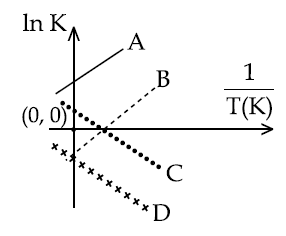## Filters

Sort by :
Clear All
Q
Engineering
91 Views   |

An ideal gas undergoes a cyclic process as shown in Figure.Heat absorbed by the system during process CA is :

• Option 1)

• Option 2)

• Option 3)

• Option 4)

As we learned   Isochoric Process - Volume is constant during the process - wherein     Isobaric process - Pressure is constant during the process - wherein      Isobaric  Isochoric  Not Defined               2 - 5  =  -3KJ                     -3 - 5 = -3KJ                         Q+w 8 = Q+3 Q= +5KJ     Option 1)       Option 2) Option 3) Option 4)
Engineering
106 Views   |

For which of the following reactions,  is equal to  ?

• Option 1)

• Option 2)

• Option 3)

• Option 4)

As we learned Molar heat capacity for isobaric process C(p) -   - wherein or     Therefore ;  Option 1)       Option 2) Option 3) Option 4)
Engineering
116 Views   |

The combustion of benzene (l) gives CO2(g) and H2O(l). Given that heat of combustion of benzene at constant volume is −3263.9 kJ mol−1 at 258 C; heat of combustion (in kJ mol−1) of benzene at constant pressure will be :

(R=8.314 JK−1 mol−1)

• Option 1)

−3267.6

• Option 2)

4152.6

• Option 3)

−452.46

• Option 4)

3260

As we learned Molar heat capacity for isobaric process C(p) -   - wherein or     For chemical reactions;          Option 1) −3267.6   Option 2) 4152.6   Option 3) −452.46   Option 4) 3260
Engineering
197 Views   |

Which of the following lines correctly show the temperature dependence of equilibrium constant, K, for an exothermic
reaction ?• Option 1)

A and D

• Option 2)

A and B

• Option 3)

B and C

• Option 4)

C and D

As we learned @1380 For exothermic reaction slope will be positive & intercept may be positive or negative for a graph of so correct graph are A & B   Option 1) A and D Option 2) A and B Option 3) B and C Option 4) C and D
Engineering
130 Views   |

Given:

Free energy change,  for the reaction     will be :

• Option 1)

−112.4 kJ mol −1

• Option 2)

−56.2 kJ mol −1

• Option 3)

−168.2 kJ mol −1

• Option 4)

−208.0 kJ mol −1

As we have learnt,

ΔG{reaction} -

- wherein

Sum of   of all product

Sum of   of all reactant

Option 1)

−112.4 kJ mol −1

Option 2)

−56.2 kJ mol −1

Option 3)

−168.2 kJ mol −1

Option 4)

−208.0 kJ mol −1

View More
Engineering
799 Views   |

fG0 at 500 K for substance ‘S’ in liquid state and gaseous state are +100.7 kcal mol −1 and +103 kcal mol−1 , respectively. Vapour pressure of liquid ‘S’ at 500 K is approximately equal to :
(R=2 cal K−1 mol−1 )

• Option 1)

0.1 atm

• Option 2)

1 atm

• Option 3)

10 atm

• Option 4)

100 atm

As we have learnt,   Δ G of equilibrium -   - wherein At Equilibrum     and     Option 1) 0.1 atm Option 2) 1 atm Option 3) 10 atm Option 4) 100 atm
Engineering
82 Views   |

For which of the following processes, ΔS  is negative ?

• Option 1)

• Option 2)

• Option 3)

• Option 4)

As we have learned Change in entropy for ideal gas in terms of C(p) - - wherein Molar heat capacity at constant pressure       for isothermal process            Option 1) This is incorrect Option 2) This is correct Option 3) This is incorrect Option 4) This is incorrect
Exams
Articles
Questions